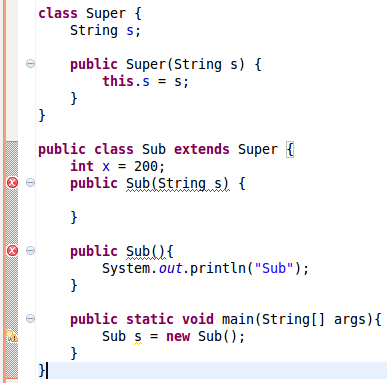# Java程序员容易犯的10个错误

## 1. Array 转 ArrayList

`List<String> list = Arrays.asList(arr);`

Arrays.asList() 会返回一个ArrayList，这是Arrays里内嵌的一个私有静态类，而并不是java.util.ArrayList类java.util.Arrays.ArrayList 有set(), get(), contains()方法，但并支持添加元素，所以大小是固定的，想要创建一个真正的ArrayList，你应该：

`ArrayList<String> arrayList = new ArrayList<String>(Arrays.asList(arr));`

## 2.检测一个Array是否包含一个元素

```Set<String> set = new HashSet<String>(Arrays.asList(arr));
return set.contains(targetValue);```

`Arrays.asList(arr).contains(targetValue);`

```for(String s: arr){
if(s.equals(targetValue))
return true;
}
return false;```

## 3. 在一个循环中移除list中的元素

```ArrayList<String> list = new ArrayList<String>(Arrays.asList("a", "b", "c", "d"));
for (int i = 0; i < list.size(); i++) {
list.remove(i);
}
System.out.println(list);```

```ArrayList<String> list = new ArrayList<String>(Arrays.asList("a", "b", "c", "d"));

for (String s : list) {
if (s.equals("a"))
list.remove(s);
}```

```ArrayList<String> list = new ArrayList<String>(Arrays.asList("a", "b", "c", "d"));
Iterator<String> iter = list.iterator();
while (iter.hasNext()) {
String s = iter.next();

if (s.equals("a")) {
iter.remove();
}
}```

## 5.使用原始类型的集合

```public static void add(List list, Object o){
}
public static void main(String[] args){
List<String> list = new ArrayList<String>();
String s = list.get(0);
}```

```Exception in thread "main" java.lang.ClassCastException: java.lang.Integer cannot be cast to java.lang.String
at ...```

## 8.Mutable vs. Immutable（可变 VS 不可变）

```String result="";
for(String s: arr){
result = result + s;
}```

## 9.父类和子类的构建```public Super(){
System.out.println("Super");
}```

## 10.”" or Constructor?

```//1. use double quotes
String x = "abc";
//2. use constructor
String y = new String("abc");```

```String a = "abcd";
String b = "abcd";
System.out.println(a == b);  // True
System.out.println(a.equals(b)); // True

String c = new String("abcd");
String d = new String("abcd");
System.out.println(c == d);  // False
System.out.println(c.equals(d)); // True```

(0)
0%

(0)
0%